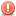CodeGuru Home VC++ / MFC / C++ .NET / C# Visual Basic VB Forums Developer.com

# Thread: Array of 100 elements ranging from 1 to 100

1.Junior MemberJoin Date
Sep 2016
Posts
22

##Array of 100 elements ranging from 1 to 100

Hello!

I'm trying to create an array of 100 elements, ranging from 1 to 100.

Code:
```int main()
{
int arr;

// For setting values to elements
for (int i = 0; i < 101; i++)
{
arr[i] = i;
}

// For getting values from elements
for (int i = 0; i < 101; i++)
{
cout << arr[i] << endl;
}

return 0;
}```
The problem with this code is that in element 0 I have 0, in element 1 I have 1, in element 2 I have 2, and so on.

I need number 1 in element 0, number 2 in element 1, number 3 in element 2, and so on. What am I missing?Reply With Quote

2.Junior MemberJoin Date
Sep 2016
Posts
22

## Re: Array of 100 elements ranging from 1 to 100

I think I found the problem. Here is the solution:

Code:
```int main()
{
int arr;

// For setting values to elements
for (int i = 0; i < 100; i++)
{
arr[i] = i + 1;
}

// For getting values from elements
for (int i = 0; i < 100; i++)
{
cout << arr[i] << endl;
}

return 0;
}```
Last edited by 2kaud; October 3rd, 2016 at 02:24 AM.Reply With Quote

3. ## Re: Array of 100 elements ranging from 1 to 100

Yep. Just a comment. When dealing with a fixed size array, IMO it is better to have a const variable to hold the number of elements in the array. That way the loops etc get the correct terminating condition and if the array size changes, only one value in the program is changed. Note that the variable needs to be const as the c++ compiler needs to know the value at compile time. Consider
Code:
```int main()
{
const int arrsize = 100;

int arr[arrsize];

// For setting values to elements
for (int i = 0; i < arrsize; i++)
arr[i] = i + 1;

// For getting values from elements
for (int i = 0; i < arrsize; i++)
cout << arr[i] << endl;

return 0;
}```
Also note that if there is only one statement following the for() then braces are not required (same for if etc).Reply With Quote

4.Elite Member Power PosterJoin Date
Jan 2006
Location
Singapore
Posts
6,765

## Re: Array of 100 elements ranging from 1 to 100Originally Posted by 2kaud
Also note that if there is only one statement following the for() then braces are not required (same for if etc).
However, you may wish to consistently put the braces even when they are not required so as to avoid having them accidentally left out should the code be changed such that braces become required, or when code is introduced with poor indentation such that it appears to be part of the loop body when it isn't.Reply With Quote

array, c++, increment####Posting Permissions

• You may not post new threads
• You may not post replies
• You may not post attachments
• You may not edit your posts
•